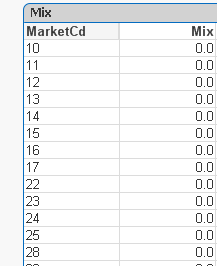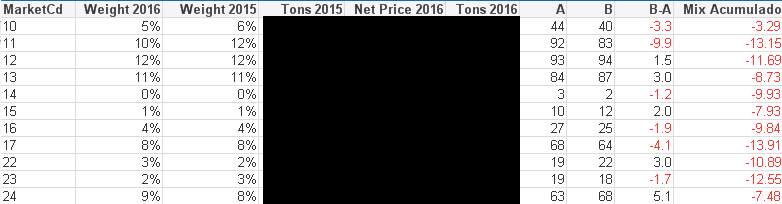# QlikView App Dev

Discussion Board for collaboration related to QlikView App Development.

Announcements
cancel
Showing results for
Did you mean:Contributor III

## Weighted Average/Sumproduct issue

Dear Qlik Community,

I've stumbled upon a rather annoying problem I can't seem to solve.

Despite finding many questions related to this and trying some solutions I've reached a dead end.

You see, I have a table with Sales: sum(Volume) and Prices: sum(Prices)/sum(sales) as expressions and Market as dimension.

Something like this:

 Market Sales Price A 577 280 B 3,879 300 C 4,582 310 D 1,242 309 E 5,793 223 F 6,901 315 G 2,420 250 Total 25,393 284

This table is for 2016, but I need two extra columns for 2015 (Sales + Price 2015). I created them as well in Qlik, no problem.

The problem is, I need to find the mix effect of one year vs another.

Now the way I do this in excel is simply sumproduct the prices 2016 * the sales volume 2015, and them divide that by the total sales volume 2015.

I'm not worried about the mix effect by market, but in TOTAL. For example, if the price is now 284, and with the weight of sales 2015, the price would be... 280, for example. That's a difference of 4 euros.

I've created the expression

Prices 2016 =   sum(Prices 2016)/sum(sales 2016)

Sales 2015 = sum (Sales 2015)

(Prices 2016 * Sales 2015)/ sum(TOTAL Sales 2015 )

What QV gives me (BELOW) is the correct expression for each market, of course. But I need the total effect, not the effect by market. However, I need to ponder each market weight for the formula to work.

As you can see, the total remains the same as Price 2016: 283.537 €

The correct value would be 283.957 € if I use sumproduct() in excel. The correct difference is 0.42 €.

I can't really find a solution. This is part of a very important report to be issued every week in the company. Could you help?

Which formula can I use to calculate something like this weighted average?  Would I remove the dimension?

What I need is a simple Sumproduct(Sales;Price) / sum(Total Sales 2015).

 Market Sales 2016 Price 2016 Sales 2015 W Average A 577 280 800 9 B 3,879 300 3,812 45 C 4,582 310 4,580 55 D 1,242 309 1,327 16 E 5,793 223 5,632 49 F 6,901 315 7,002 86 G 2,420 250 2,490 24 Total 25,393 284 25,643 284

If this is not the correct method, could you give me any alternatives for calculating such an average?

Please bear in mind I'm really a noviceMariana.

5 RepliesMVP

Can you try this:

Sum(Aggr((Prices 2016 Expression * Sales 2015 Expression)/ sum(TOTAL Sales 2015 Expression), Market))

Make sure to list out the complete expressions within the Aggr() function, as it doesn't work with Column Labels (in case you are using that)Contributor III
Author

Dear Sunny,

Thank you so much for helping me.

However the expression is giving me 0, and I have no idea why?

I did try a similar expression with aggr, but I wasn't sure it was correct before and it gave me the same result.

Is there any possibility to get a similar sumproduct() result as we do in excel?Thanks again,

Mariana.MVP

Would you be able to share a sample to help you better here?Contributor III
Author

Hi Sunny,

Unfortunately, I have one of those "data is confidential" problems.

I can share the expressions, no problem.

I managed to find the correct number by multiplying the weight in % of the volume 2015 by the price 2016.

If I cummulate that expression, I get the correct value I was talking about above!

Below is the accurate chart. The values in black are real, so I cannot share them. So I multiplied each weight (year) by the price 2016 and got expressions A and B. The last column is the same as B-A but cummulated.

My problem is I wanted this fairly automatic in order to get a bar chart and put it on a report.

Seems to me it needs to be manual, nonetheless.Many thanks,

MarianaMVP